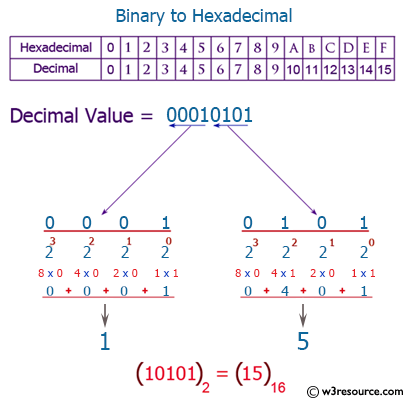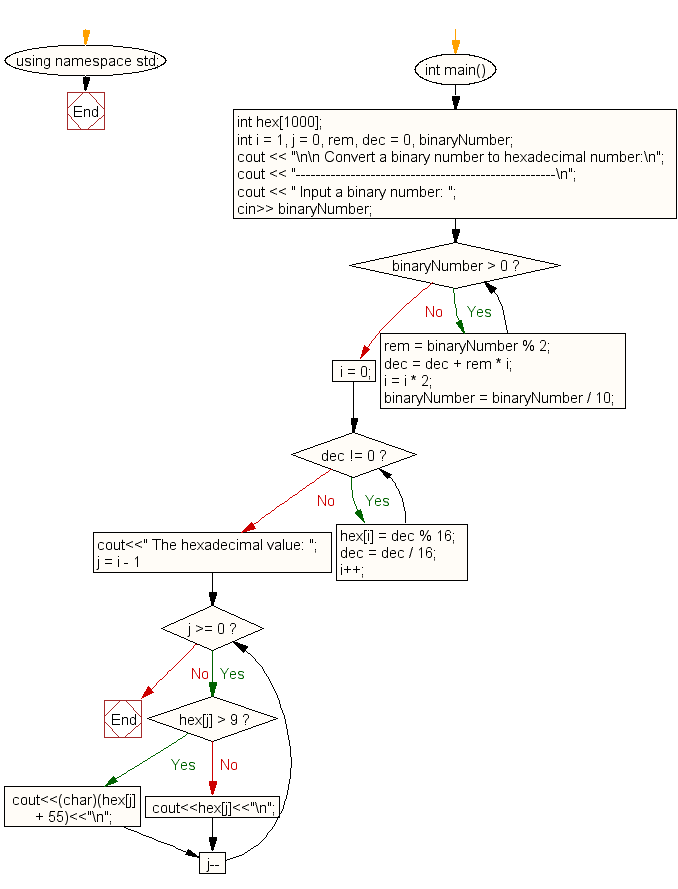﻿ C++ Exercises: Convert a binary number to hexadecimal number - w3resource# C++ Exercises: Convert a binary number to hexadecimal number

## C++ For Loop: Exercise-74 with Solution

Write a program in C++ to convert a binary number to hexadecimal number.

Pictorial Presentation:Sample Solution:-

C++ Code :

``````#include <iostream>
#include <math.h>
using namespace std;

int main()
{
int hex;
int i = 1, j = 0, rem, dec = 0, binaryNumber;
cout << "\n\n Convert a binary number to hexadecimal number:\n";
cout << "----------------------------------------------------\n";
cout << " Input a binary number: ";
cin>> binaryNumber;
while (binaryNumber > 0)
{
rem = binaryNumber % 2;
dec = dec + rem * i;
i = i * 2;
binaryNumber = binaryNumber / 10;
}
i = 0;
while (dec != 0)
{
hex[i] = dec % 16;
dec = dec / 16;
i++;
}
cout<<" The hexadecimal value: ";
for (j = i - 1; j >= 0; j--)
{
if (hex[j] > 9)
{
cout<<(char)(hex[j] + 55)<<"\n";
}
else
{
cout<<hex[j]<<"\n";
}
}
}
``````

Sample Output:

``` Convert a binary number to hexadecimal number:
----------------------------------------------------
Input a binary number: 1011
The hexadecimal value: B
```

Flowchart:C++ Code Editor:

Contribute your code and comments through Disqus.

What is the difficulty level of this exercise?

﻿

## C++ Programming: Tips of the Day

What is a smart pointer and when should I use one?

This answer is rather old, and so describes what was 'good' at the time, which was smart pointers provided by the Boost library. Since C++11, the standard library has provided sufficient smart pointers types, and so you should favour the use of std::unique_ptr, std::shared_ptr and std::weak_ptr.

There was also std::auto_ptr. It was very much like a scoped pointer, except that it also had the "special" dangerous ability to be copied - which also unexpectedly transfers ownership.

It was deprecated in C++11 and removed in C++17, so you shouldn't use it.

```std::auto_ptr<MyObject> p1 (new MyObject());
std::auto_ptr<MyObject> p2 = p1; // Copy and transfer ownership.
// p1 gets set to empty!
p2->DoSomething(); // Works.
p1->DoSomething(); // Oh oh. Hopefully raises some NULL pointer exception.
```

Ref : https://bit.ly/3mc9GHE